# HSSlive: Plus One & Plus Two Notes & Solutions for Kerala State Board

## Tuesday, June 28, 2022BSEB Class 9 Maths Chapter 8 Quadrilaterals Ex 8.1 Textbook Solutions PDF: Download Bihar Board STD 9th Maths Chapter 8 Quadrilaterals Ex 8.1 Book Answers

## Bihar Board Class 9th Maths Chapter 8 Quadrilaterals Ex 8.1 Books Solutions

 Board BSEB Materials Textbook Solutions/Guide Format DOC/PDF Class 9th Subject Maths Chapter 8 Quadrilaterals Ex 8.1 Chapters All Provider Hsslive

2. Click on the Bihar Board Class 9th Maths Chapter 8 Quadrilaterals Ex 8.1 Answers.
3. Look for your Bihar Board STD 9th Maths Chapter 8 Quadrilaterals Ex 8.1 Textbooks PDF.

Find below the list of all BSEB Class 9th Maths Chapter 8 Quadrilaterals Ex 8.1 Textbook Solutions for PDF’s for you to download and prepare for the upcoming exams:

## BSEB Bihar Board Class 9th Maths Solutions Chapter 8 Quadrilaterals Ex 8.1

Question 1.
The angles of quadrilateral are in the ratio 3 : 5 : 9 : 13. Find all the angles of the quadrilateral.
Solution:
Let the angles be (3x)°, (5x)°, (9x)° and (13x)°
Then, 3x + 5x + 9x + 13x = 360
⇒ 30x = 360
⇒ x = 36030 = 12
∴ The angles are (3 x 12)°, (5 x 12)°, (9 x 12)° and (13 x 12)° i.e., 36°, 60°, 108° and 156°.

Question 2.
If the diagonals of a parallelogram are equal, then show that it is a rectangle.
Solution:
A parallelogram ABCD in which AC = BD.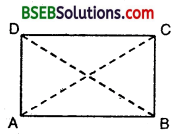To prove : ABCD is a rectangle.
Proof :
In ∆s ABC and DCB, we have
AB = DC [Opp.sides of a ||gm]
BC = BC [Common]
and, AC = DB [Given]
∴ By SSS criterion of congruence.
∆ ABC ≅ ∆ DCB
⇒ ∠ABC = ∠DCB … (1)
[Corresponding parts of congruent triangles are equal]
But AB || DC and BC cuts them.
∴ ∠ACB + ∠DCB = 180° … (2)
⇒ 2∠ABC = 180° [Sum of consecutive interior angles is 180°]
⇒ ∠ABC = 90°
Thus, ∠ABC = ∠DCB= 90°
⇒ ABCD is a parallelogram one of whose angle is 90°.
Hence, ABCD is a rectangle.

Question 3.
Show that if the diagonals of a quadrilateral bisect each other at right angles, then it is a rhombus.
Solution:
A quadrilateral ABCD in which the diagonals AC and BD intersect at O such that AO = OC,
BO = OD and AC ⊥ BD.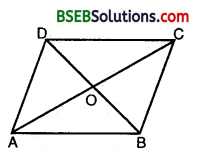To prove : ABCD is a rhombus.
Proof:
Since the diagonals AC and BD of quadrilateral ABCD bisect each other at right angles.
∴ AC is the perpendicular bisector of the segment BD.
⇒ A and C both are equidistant from B and D.
⇒ AB = AD and CB = CD … (1)
Also, BD is the perpendicular bisector of line segment AC.
⇒ B and D both are equidistant from A and C.
⇒ AB = BC and AD = DC … (2)
From (1) and (2), we get
AB = BC = CD = AD
Thus, ABCD is a quadrilateral whose diagonals bisect each other at right angles and all four sides are equal.
Hence, ABCD is a rhombus. .
Second Proof: First we shall prove that ABCD is a ||gm
In ∆s AOD and COB, we have
AO = OC
OD = OB [Given]
∠AOD = ∠COB [∵ertically opp. angles]
By SAS criterion of congruence,
∆ AOD ≅ ∆ COB
⇒ ∠OAD = ∠OCB … (1)
[Corresponding parts of congruent triangles are equal] Now, line AC intersects AD and BC at A and C respectively such that
i.e., alternate interior angles are equal.
AB || CD Hence, ABCD is a parallelogram.
Now, we shall prove that ||gm ABCD is a rhombus.
In ∆s AOD and COD, we have
OA = OC [Given]
∠AOD = ∠COD [Both are right angles]
OD = OD [Common]
∴By SAS criterion of congruence
∆ AOD ≅ ∆ COD
⇒ AD = CD … (2)
[Corresponding parts of congruent triangles are equal] Now, ABCD is a ||gm [Proved above]
⇒ AB = CD and AD = BC
[Opp. sides of a ||gm are equal] ⇒ AB = CD = AD = BC [Using (2)]
Hence, quadrilateral ABCD is a rhombus.

Question 4.
Show that the diagonals of a square are equal and bisect each other at right angles.
Solution:
Given : A square ABCD.
To prove : AC = BD, AC ⊥ BD and OA = OC, OB = OD.Proof:
Since ABCD is a square. Therefore, AB || DC and AD || BC.
Now, AB || DC and transversal AC intersects them at A and C respectively.
∴ ∠BAC = ∠DCA
[Alternate interior angles are equal]
⇒ ∠BAO =∠DCO … (1)
Again, AB || DC and BD intersects them at B and D respectively.
[∵ Alternate interior angles are equal] … (2)
Now, in ∆s AOB and ∠COD, we have
∠BAO = ∠DCO [From (1)]
AB = CD [Opposite sides of a ||gm are equal]
and, ∠ABO = ∠CDO [From (2)]
By ASA congruence criterion
∆ AOB ≅ ∆ COD
⇒ OA = OC and OB = OD
[Corresponding parts of congruent As are equal] Hence, the diagonals bisect each other.
In ∆s ADB and BCA, we have
AD = BC [Sides of a square are equal]
∠BAD = ∠ABC [Each equal to 90°}
and, AB = BA [Common]
∴ By SAS criterion of congruence
⇒ AC = BD
[∵ Corresponding parts of congruent As are equal]
Hence, the diagonals are equal.
Now in As AOB and AOD, we have OB = OD
[∵ Diagonals of ||gm bisect each other]
[∵ Sides of a square are equal]
and, AO = AO [Common]
∴ By SSS criterion of congruence
∆ AOB ≅ ∆ AOD
⇒ ∠AOB – ∠AOD
[Corresponding parts of congruent ∆s are equal]
But ∠AOB + ∠AOD = 180°
∴∠AOB = ∠AOD = 90°
⇒ AO ⊥ BD
⇒ AC ⊥ BD .
Hence, diagonals intersect at right angles.

Question 5.
Show that if the diagonals of a quadrilateral are equal and bisect each other at right angles, then it is a square.
Solution:
Given : A quadrilateral ABCD in which the diagonals AC = BD, AO = OC, BO = OD and AC ⊥ BD.
To prove : Quadrilateral ABCD is a square.
Proof :
First, we shall prove that ABCD is a parallelogram.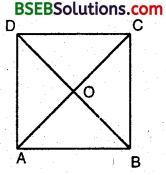In ∆s AOD and COB, we have AO = OC OD = OB
∠AOD = ∠COB [∵Vertically opp. angles]
By SAS criterion of congruence,
∆ AOD ≅ ∆ COB
[Corresponding parts of congruent triangles are equal] Now, line AC intersects AD and BC at A and C respectively such that
i.e., alternate interior angles are equal.
Similarly, AB || CD
Hence, ABCD is a parallelogram.
Now, we shall prove that it is a square.
In ∆s AOB and ∠AOD, we have
AO = BO [Common]
∠AOB = ∠AOD [Each = 90°, given]
and, OB = OD [∵ Diagonals of a ||gm bisect each other]
∴By SAS criterion of congruence
∆ ABD ≅ ∆ BAC
[Corresponding parts of congruent triangles are equal]
But AB = CD and AD = BC
[Opp. sides of a ||gm are equal]
∴ AB = BC = CD = AD … (2)
Now, in ∆s ABD and BAC, we have
AB = BA [Common]
AD = BC [Opp. sides of a ||gm are equal] and, BD = AC [Given]
∴ By SSS criterion of congruence
∆ ABD ≅ ∆ BAC
⇒ ∠DAB = ∠CBA [Corresponding parts of congruent As are equal]

Question 6.
Diagonal AC of a nparallelogram ABCD bisects ∠A see figure). Show that(i) it bisects ∠C also,
(ii) ABCD is a rhombus.
Solution:
(i) Given : A parallelogram ABCD in which diagonal AC bisects ∠A.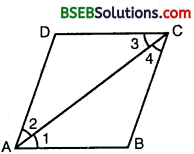To prove : That AC bisects ∠C.
Proof:
Since ABCD is a ||gm. Therefore, AB || DC.
Now, AB || DC and AC intersects them
∴ ∠1 = ∠3 … (1) [Alternate interior angles]
Again, AD || BC and AC intersects them
∴ ∠2 = ∠4 … (2) [Alternate interior angles]
But it is given that AC is the bisector of ∠A.
∴ ∠1 = ∠2 … (3)
From (1), (2) and (3), we have ∠3 = ∠4
Hence, AC bisects ∠C.

(ii) To prove : That ABCD is a rhombus.
From part (i) : (1), (2) and (3) give ∠1 = ∠2 = ∠3 = ∠4 Now in ∆ ABC,
∠1 = ∠4
AB = BC [Sides opp. to equal angles in a ∆ are equal]
Similarly, in ∆ ADC, we have
Also, ABCD is a ||gm
∴ AB = CD, AD = BC
Combining these, we get
AB = BC = CD = DA
Hence, ABCD is a rhombus.

Question 7.
ABCD is a rhombus. Show that diagonal AC bisects ∠A as well as ∠C and diagonal BD bisects ∠B as well as ∠D.
Solution:
Given : A rhombus ABCD.To prove that (i) Diagonal t AC bisects ∠A as well ∠C.
(ii) Diagonal BD bisects ∠B as well as ∠D.
Proof:
[Sides of a rhombus are equal]
⇒ ∠DAC = ∠DCA …(1)
[Angles opp. to equal sides of a triangle are equal]
Now AB || DC and AC intersects them
∠BCA = ∠DAC …(2) [Alternate angles]
From (1) and (2), we have
∠DCA = ∠BCA
⇒ AC bisects ∠C
In ∆ ABC, AB = BC [Sides of a rhombus are equal]
⇒ ∠BCA = ∠BAC …(3)
[Angles opp. to equal sides of a triangle are equal]
From (2) and (3), we have
∠BAC = ∠DAC
⇒ AC bisects ∠A
Hence, diagonal AC bisects ∠A as well as ∠C.
Similarly, diagonal BD bisects ∠B as well as ∠D.

Question 8.
ABCD is a rectangle in which diagonal AC bisects ∠A as well as ∠C. Show that : (i) ABCD is a square (ii) diagonal BD bisects ∠B as well as ∠D.
Solution:
Given : ABCD is a rectangle in which diagonal AC bisects ∠A as well as ∠C.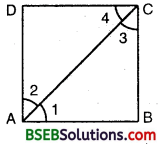To prove that (i) ABCD is a square
(ii) diagonal BD bisects ∠B as well as ∠D.
Proof:
(i) Since AC bisects ∠A as well as ∠C in the rectangle ABCD.
∴ ∠1 = ∠2 = ∠3 = ∠4 [∵ Each = 90°2 = 45°]
∴ In ∆ ADC, ∠2 = ∠4
⇒ AD = CD [Sides opposite to equal angles]
Thus, the rectangle ABCD is a square.

(ii) In a square, diagonals bisect the angles.
So, BD bisects ∠B as well as ∠D.

Question 9.
In parallelogram ABCD, two points P and Q are taken dn diagonal BD such that DP = BQ (see figure). Show that:(i) ∆ APD ≅ ∆ CQB
(ii) AP = CQ
(iii) ∆ AQB ≅ ∆ CPD
(iv) AQ = CP
(v) APCQ is a parallelogram
Solution:
ABCD is a parallelogram. P and Q are points on the diagonal BD such that DP = BQ.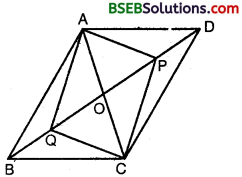To prove :
(i) ∆ APD ≅ ∆ CQB
(ii) AP = CQ
(ii) ∆ AQB ≅ ∆ CPD
(iv) AQ = CP
(v) APCQ is a parallelogram
Construction : Join AC to meet BD in O.
Proof:
We know that the diagonals of a parallelogram bisect each other. Therefore, AC and BD bisect each other at O.
OB = OD
But BQ = DP [Given]
⇒ OB – BQ = OD – DP ⇒ OQ = OP
Thus, in quadrilateral APCQ diagonals AC and PQ are such that OQ = OP and OA = OC. i.e., the diagonals AC and PQ bisects each other.
Hence, APCQ is a parallelogram, which prove the (v) part.
(i) In ∆s APD and CQB, we have
AD = CB [Opp. sides of a ||gm ABCD]
AP = CQ [Opp. sides of a ||gm APCQ]
DP = BQ [Given]
∴ By SSS criterion of congruence
∆ APD ≅ ∆ CQB

(ii) AP = CQ [Opp. sides of a ||gm APCQ]

(iii) In As AQB and CPD, we have
AB = CD [Opp. sides of a ||gm ABCD]
AQ = CP [Opp. sides of a ||gm APCQ]
BQ = DP [Given]
∴ By SSS criterion of congruence
∆ AQB ≅ ∆ CPD

(iv) AQ = CP [Opp. sides of a ||gm APCQ]

Question 10.
ABCD is a parallelogram and AP and CQ are perpendiculars fi-om vertices A and C on diagonal BD respectively (see figure). Show that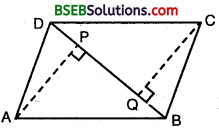(i) ∆ APB ≅ ∆ CQD
(ii) AP = CQ
Solution:
(i) Since ABCD is a parallelogram. Therefore,
DC || AB.
Now, DC || AB and transversal BD intersects them at B and D.
∴ ∠ABD = ∠BDC [Alternate interior angles]
Now, in As APB and CQD, we have
∠ABP = ∠QDC [∵ ∠ABD = ∠BDC]
∠APB = ∠CQD [Each = 90°]
and, AB = CD [Opp. sides of a ||gm]
∴ By AAS criterion of congruence
∆ APB ≅ ∆ CQD

(ii) Since ∆ APB ≅ ∆ CQD
∴ AP = CQ
[∵ Corresponding parts of congruent triangles are equall

Question 11.
In ∆ ABC and ∆ DEF, AB = DE, AB || DE, BC = EF and. BC || EF. Vertices A, B and C are joined to vertices D, E and F respectively (see figure). Show that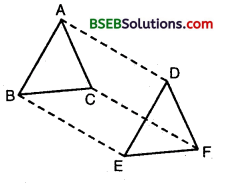(i) quadrilateral ABED is a parallelogram
(ii) quadrilateral BEFC is a parallelogram
(iv) quadrilateral ACFD is a parallelogram
(v) AC = DF
(vi) ∆ ABC ≅ ∆ DEF.
Solution:
Given : Two ∆s ABC and DEF such that AB = DE and AB || DE. Also BC = EF and BC || EF.
To prove that: (i) quadrilateral ABED is a parallelogram.
(ii) quadrilateral BEFC is a parallelogram.
(iv) quadrilateral ACFD is a parallelogram.
(v) AC || DF and AC = DF
(vi) ∆ ABC = ∆ DEF.
Proof: (i) Consider the quadrilateral ABED
We have, AB = DE and AB || DE
⇒ One pair of opposite sides are equal and parallel.
⇒ ABED is a parallelogram.

(ii) Now, consider quadrilateral BEFC, we have
BC = EF and BC || EF
One pair of opposite sides are equal and parallel.
⇒ BEFC is a parallelogram.

(iii) Now, AD = BE and AD || BE … (1) [∵ ABED is a ||gm]
and CF = BE and CF || BE … (2) [∵ BEFC is a ||gm]
From (1) and (2), we have

⇒ One pair of opposite sides are equal and parallel
⇒ ACFD is a parallelogram.

(v) Since ACFD is a parallelogram.
∴ AC = DF [Opp. sides of a ||gm ACFD]

(vi) In ∆s ABC and DEF, we have
AB = DE [Opp. sides of a ||gm ABED]
BC = EF [Opp. sides of a ||gm BEFC]
and, CA = FD [Opp. sides of ||gm ACFD]
∴ By SSS criterion of congruence ∆ ABC ≅ ∆ DEF.

Question 12
ABCD is a trapezium in which AB || CD and AD = BC (see figure). Show that(i) ∠A = ∠B
(ii) ∠C = ∠D
(iii) ∆ ABC ≅ ∆ BAD
(iv) diagonal AC = diagonal BD
Solution:
Given : ABCD is a trapezium in which AB || CD and AD = BC.
To prove that:
(i) ∠A = ∠B
(ii) ∠C = ∠D
(iii) ∆ ABC ≅ ∆ BAD
(iv) diagonal AC = diagonal BD.
Construction : Produce AB and draw a line CE || AD.
Proof:
(i) Since AD || CE and transversal AE cuts them at A and E respectively.
∴ ∠A + ∠E = 180° … (1)
Since AB || CD and AD || CE. Therefore, AECD is a parallelogram.
BC = CE [∵ AD = BC (given)]
Thus, in A BCE, we have BC = CE
⇒ ∠CBE = ∠CEB
⇒ 180 – ∠B = ∠E
⇒ 180 – ∠E = ∠B
From (1) and (2), we get ∠A = ∠B

(ii) Since ∠A = ∠B ⇒ ∠BAD = ∠ABD
⇒ 180° – ∠BAD = 180° – ∠ABD
⇒ ∠D = ∠C i.e., ∠C = ∠D.

(iii) In ∆s ABC and BAD, we have
AB = BA [Common]
∠A = ∠B [Proved]
∴ By SAS criterion of congruence ∆ ABC ≅ ∆ BAD

(iv) Since ∆ ABC = ∆ BAD
∴ AC = BD
[Corresponding parts of congruent triangles are equal]

## Bihar Board Class 9th Maths Chapter 8 Quadrilaterals Ex 8.1 Textbooks for Exam Preparations

Bihar Board Class 9th Maths Chapter 8 Quadrilaterals Ex 8.1 Textbook Solutions can be of great help in your Bihar Board Class 9th Maths Chapter 8 Quadrilaterals Ex 8.1 exam preparation. The BSEB STD 9th Maths Chapter 8 Quadrilaterals Ex 8.1 Textbooks study material, used with the English medium textbooks, can help you complete the entire Class 9th Maths Chapter 8 Quadrilaterals Ex 8.1 Books State Board syllabus with maximum efficiency.

## FAQs Regarding Bihar Board Class 9th Maths Chapter 8 Quadrilaterals Ex 8.1 Textbook Solutions

#### Can we get a Bihar Board Book PDF for all Classes?

Yes you can get Bihar Board Text Book PDF for all classes using the links provided in the above article.

## Important Terms

Bihar Board Class 9th Maths Chapter 8 Quadrilaterals Ex 8.1, BSEB Class 9th Maths Chapter 8 Quadrilaterals Ex 8.1 Textbooks, Bihar Board Class 9th Maths Chapter 8 Quadrilaterals Ex 8.1, Bihar Board Class 9th Maths Chapter 8 Quadrilaterals Ex 8.1 Textbook solutions, BSEB Class 9th Maths Chapter 8 Quadrilaterals Ex 8.1 Textbooks Solutions, Bihar Board STD 9th Maths Chapter 8 Quadrilaterals Ex 8.1, BSEB STD 9th Maths Chapter 8 Quadrilaterals Ex 8.1 Textbooks, Bihar Board STD 9th Maths Chapter 8 Quadrilaterals Ex 8.1, Bihar Board STD 9th Maths Chapter 8 Quadrilaterals Ex 8.1 Textbook solutions, BSEB STD 9th Maths Chapter 8 Quadrilaterals Ex 8.1 Textbooks Solutions,
Share: